HistoryMathematiciansAll formulasNum. approx.SoftwaresMisc. math.DigitsPoetryPapers/videosDelirium !Pi-DayImagesMusicLinksBibliography Boris Gourévitch The world of Pi - V2.57 modif. 13/04/2013HomeVersion historyGuestbookWho I amSome pictures (fr)AcknowledgementsLast modificationsContact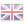### 10 Introduction to factorials and combinations

What is very interesting, is that we will always be able to observe the same kind of constant even with the introduction of an equivalent combination or factorial (as long as we stay in a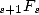), this is why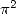and the logarithms will represent the essential results given by simple hypergeometric. On the other hand, as soon as we introduce a squared combination for example, we will obtain different kind, something to do with the gamma function most of the time (and so is! but of a less obvious way).

#### 10.1 A first example

The introduction of the combination is justified by several very similar series with or without the presence of combinations. For example,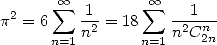(Euler)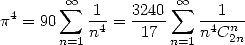(Comtet 1974)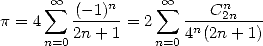(362)

Which means that in fact as soon as we find a result to this function, we have a little hope to find the same kind of result with a combinations with a central binomial coefficient for example. It's simply due to the form quite close to generators functions of those series (1211).

#### 10.2 Umbral calculus

We can establish a link betweens the series with combinations (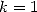more precisely) and the series without (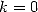) with the help of the acceleration of the convergence by Euler's method. This is a particular case of the formula for "finite differences" which is the discrete version of Taylor's expansion (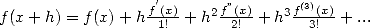). It's the theory known under the name of ”Umbral calculus”, whose origines goes back to Sylvester (1814-1897). The acceleration method by Euler consist to replacing the sum of a serie by the sum of the difference in terms of the serie.

More precisely, we choose an alternating serie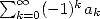and we calculate the finite difference of orderfor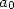: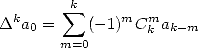(363)

then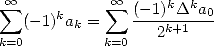(364)

The trick, it's that the first term is sometime written as combinations or factorial ratio and other symbols of Pochammer ! Application :

We know that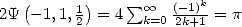. Hence we have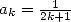.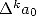being quite delicate to calculate directly, we let the function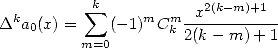(365)

We are going to look for the value inof course. And we calculate it !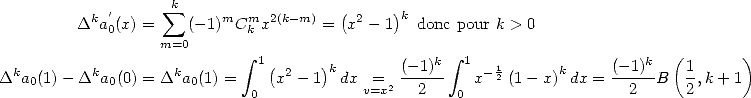where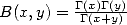is the complete Bêta function which is worth in this case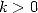integers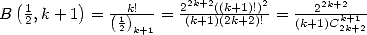(interesting...).

From which we obtain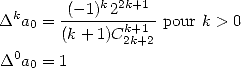and hence according to the formula 364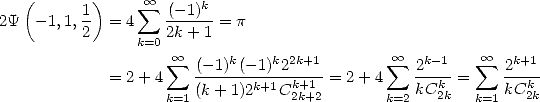Unfortunatly, I have not found how to generalise this method... However, we have still the more general formula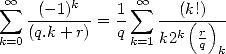(369)

or even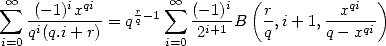(370)

where B is an incomplete Bêta , if I'm not mistaken...

Similarly, by using the link between the incomplete bêta and the hypergeometric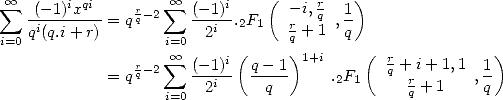where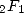is the hypergeometric function.

Basicly, the introduction to factorials can be interesting, very interesting....